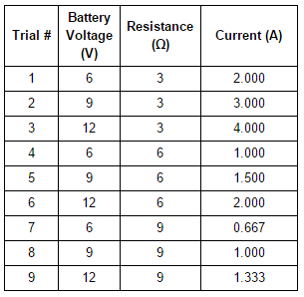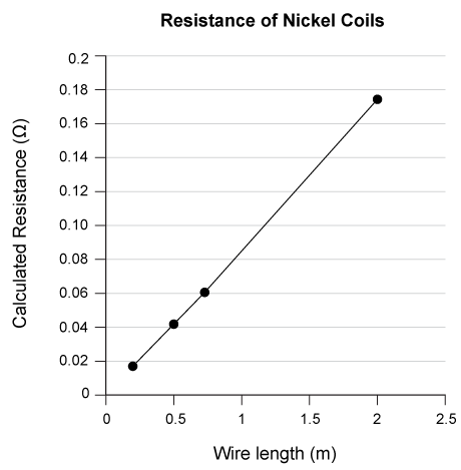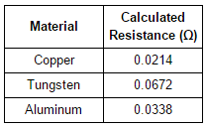Click text to edit
Add a note to this section

Passage Tools
SHOW HIDE
• Flag

Electricity can be defined as the movement of electrons. Three of the most important concepts to understand in order to manipulate electricity to perform work are voltage, current, and resistance.

Voltage (measured in volts (V)) describes the amount of potential energy between two points on a circuit and is created by a difference in charge between those two points.

Current (measured in Amperes (A)) is the rate at which electrons flow through a circuit. A rate of one ampere is equivalent to 1 coulomb (a standard unit of charge) per second.

Resistance (measured in ohms (Ω)) is a measurement of how much a material resists the passage of current through the material. Materials with high resistance are referred to as insulators, while materials with low resistance are referred to as conductors.

Students in a physics course conducted several experiments to investigate the relationship between these three electrical properties.

Experiment 1

Students were provided with a variety of batteries, resistors (electrical components that resist the flow of current), and an ammeter (a device to measure current flow), along with wire and connectors. The students constructed circuits based on the circuit diagram below and measured the current in each circuit. Table 1 shows their results.

Table 1Experiment 2

To further study the property of resistance, students replaced the resistor in their circuit with coils of nickel wire of various lengths. Students used a variable power supply to adjust voltage until current was equal to 1 A. They then used the relationship between voltage, current, and resistance determined in Experiment 1 to calculate the resistance of the wire coil. Their results are graphed in Figure 2.

Figure 2Experiment 3

Students repeated the procedure from Experiment 2 using 1 meter wire coils of a variety of other metals. Their results are given in Table 2.

Table 2Choose the option that best answers the question.

Experiments 1-3 were completed in a classroom at 20°C. During the previous school year, the air conditioning was broken, so the same lab was completed at 28°C. It is known that conductivity of metals decreases as temperature increases. How would the higher classroom temperature have affected the voltage required to reach 1 A in Experiment 2?

##### Title

Electricity can be defined

Correct

Easy